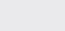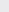Course Content

0/4

0/3

0/1

0/2

0/1

0/4

0/3

0/5

0/3

0/5

0/5

0/1

0/6

0/2

0/4

0/5

17.3 Change of Base of Logarithms 对数的换底公式

1.

Given log3 = m, log2 = n, prove that=.

2.

If log= a, state logx in terms of a.

1.5a
3.

Given that log3 = a and log7 = b. State log56 in terms of a and b.4.

Show that logxy = 2logx + 2logy. Hence or otherwise, find the values of x and y that satisfies the equation logxy = 10 and=.

16, 64
5.

If x=

*** QuickLaTeX cannot compile formula:
\log_3{{\frac{16}{9}}

*** Error message:
Missing } inserted.
leading text: $\log_3{{\frac{16}{9}}$



, y=and z=, find the values of

*** QuickLaTeX cannot compile formula:
\log_3{{\frac{16}{9}}

*** Error message:
Missing } inserted.
leading text: $\log_3{{\frac{16}{9}}$



, y=和 z=，求值：

(a)+– z

(b) 2x + y + 2z

16, 64
6.

If a=2and b=2, find the value of 2a + b9

7.

8.

If,are the roots of 3(logx)2 – 2logx + 1 = 0, form a quadratic equation which roots are log, log.,是方程式 3(logx)2 – 2logx + 1 = 0 的根, 试作一个一元二次方程式，使它的两个根是 log, log.

3x+ 5x + 3 = 0